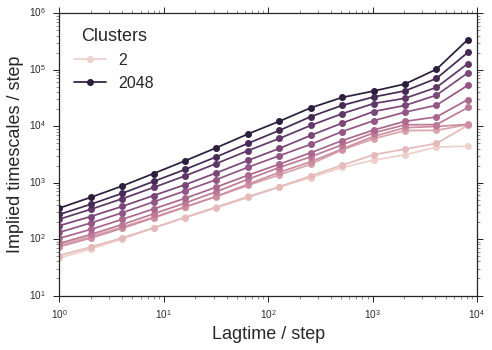# Easy parallelization with GNU parallel

There are an abundance of “scientific workflow” frameworks, job schedulers, queuing systems, MPI implementations, and language-level primitives for multiprocessing. Sometimes, though, you just have to do a task a bunch of times. Here, I introduce GNU `parallel` for a simple task and a more advanced task.

### Convert files to `png`

Maybe you just rendered a bunch of images with VMD. You try to insert them into your powerpoint presentation, but the files are `tga`. You can convert them with ImageMagick’s `convert` program. Instead of running this utility by hand each time, you can do some fancy command-line trickery:

``````find . -name "*.tga" -exec convert {} {}.png \;
``````

This works, but can be improved with `parallel`

``````find . -name "*.tga" | parallel convert {} {.}.png
``````

This method offers two advantages:

1. This will run the conversion task in parallel, exploiting your multi-core CPU

2. The new filenames will end in `.png` instead of `.tga.png`

### Run a grid search over parameters

tICA is a powerful algorithm for discovering “reaction coordinates” from molecular dynamics datasets. It’s a linear algorithm, but recent advances show how the kernel trick can be used to learn non-linear coordinates. Approximations will probably work well here. Suppose we want to calculate the tICA timescales over a grid of (`lag-time`, `degree-of-approximation`) parameter values. This is “embarrassingly parallel”. We can write our script to exploit some more advanced features of `parallel`.

``````\$ parallel echo ::: `seq 1 2` ::: a c
1 a
1 c
2 a
2 c
``````

`parallel` will automatically do the “outer product” of argument lists, separated by `:::`. Below, we use one script to both generate and consume argument lists. This script uses MSMBuilder to fit multiple tICA models.

``````#!/usr/bin/env python
# Usage:
# parallel ./tica.py ::: `./tica.py --1` ::: `./tica.py --2`

n_clusters = [2 ** i for i in range(1, 13)]
lag_times = [2 ** i for i in range(14)]

import sys
if sys.argv == '--1':
for nc in n_clusters:
print(nc)
sys.exit(1)
if sys.argv == '--2':
for lt in lag_times:
print(lt)
sys.exit(2)

nc = int(sys.argv)
lt = int(sys.argv)
components = min(10, nc)

from msmbuilder.io import load_trajs, save_generic
from msmbuilder.decomposition import tICA

# Load input trajectories, precomputed
# These have different numbers of features
meta, ftrajs = load_trajs("ftrajs-{}".format(nc))
tica = tICA(n_components=components, lag_time=lt, kinetic_mapping=True)
tica.fit(ftrajs.values())
res = {'lagtime': lt, 'clusters': nc}
for i in range(components):
res['timescale_{}'.format(i)] = tica.timescales_[i]
save_generic(res, 'tica-{}-{}.pickl'.format(nc, lt))
``````

I also include code for combining the results into one dataset in the same script by adding the additional clause to argument parsing:

``````import pandas as pd
from msmbuilder.io import load_generic
if sys.argv == '--combine':
results = []
for nc in n_clusters:
for lt in lag_times:
results += [load_generic('tica-{}-{}.pickl'.format(nc, lt))]
df = pd.DataFrame(results)
df.to_pickle('tica-timescales.pandas.pickl')
sys.exit(3)
``````